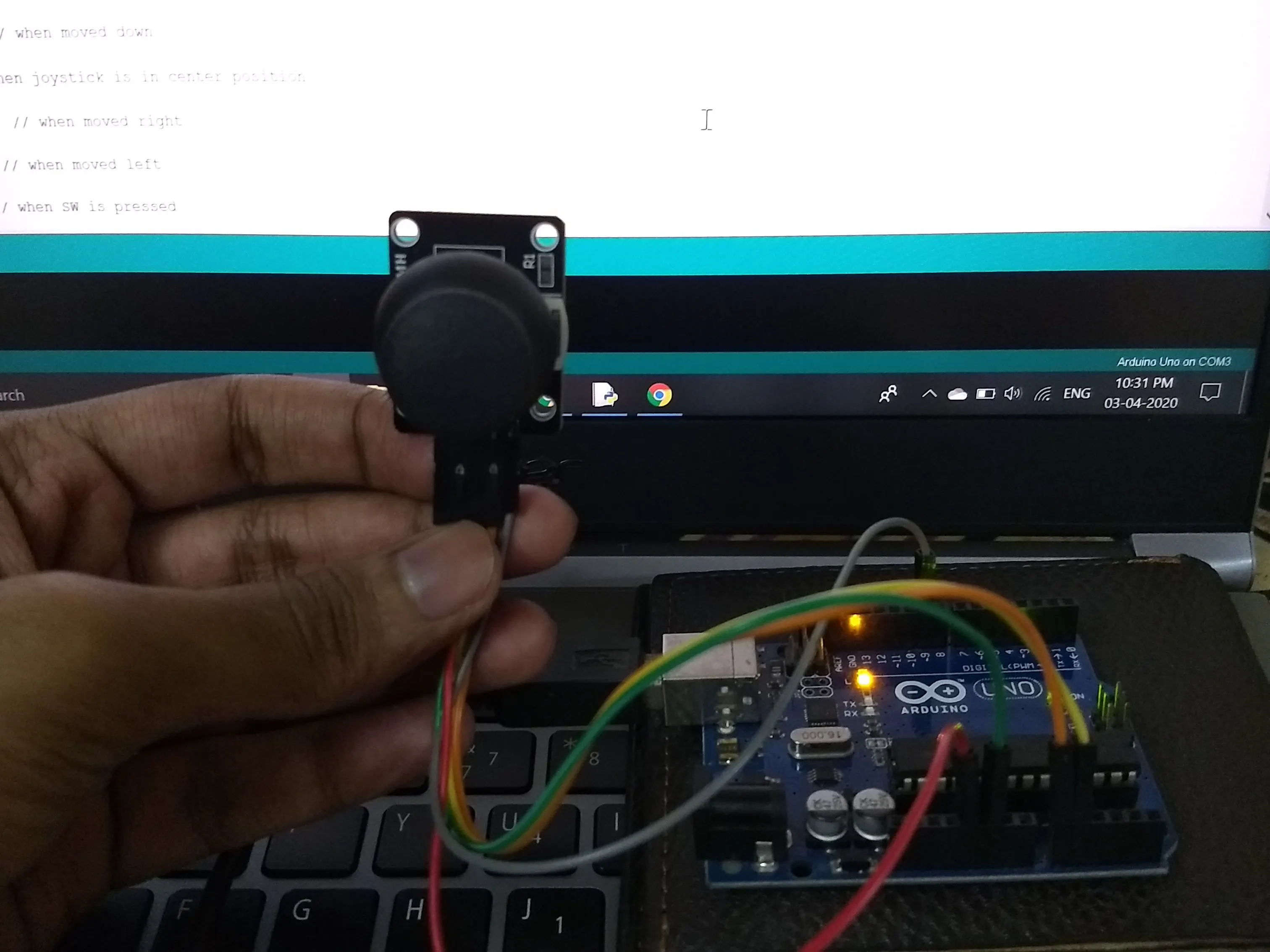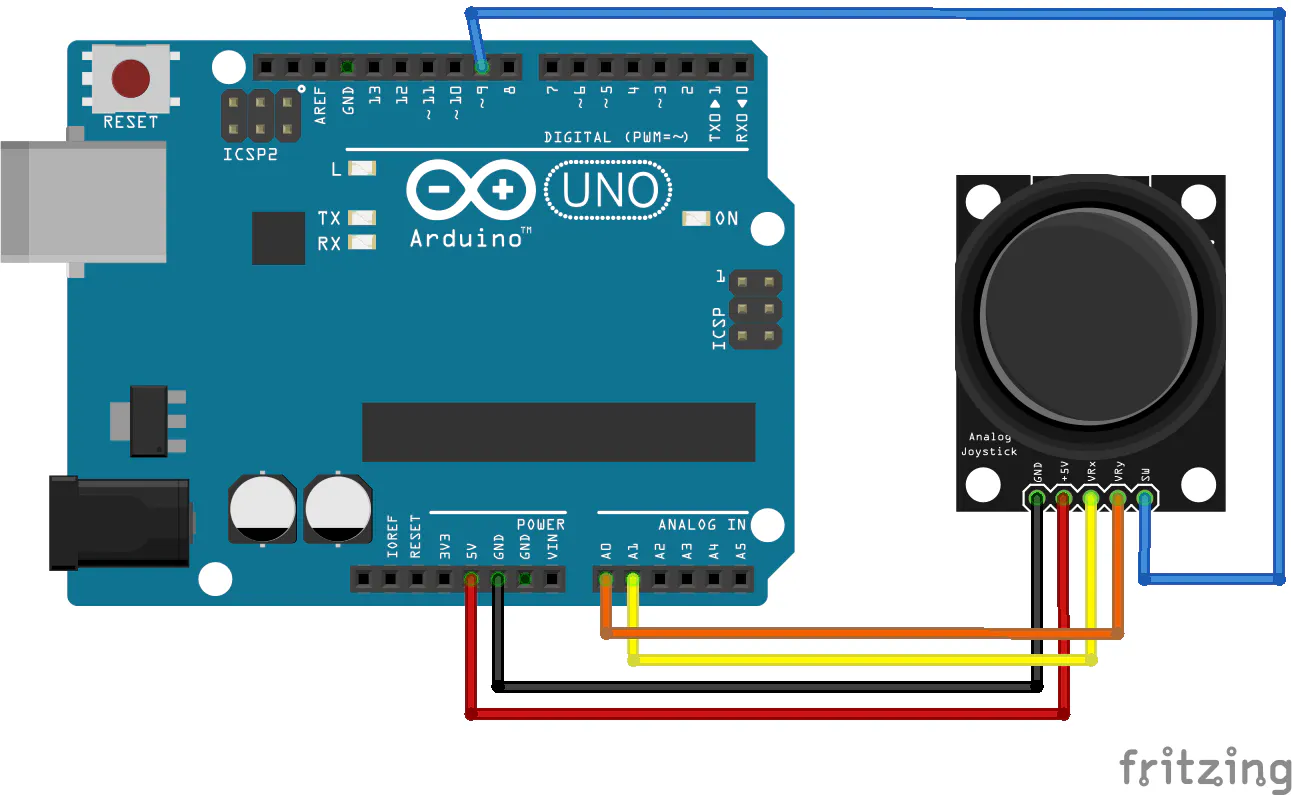# Joystick controlled mouse

Controls cursor using a simple joystick

BeginnerFull instructions provided5,448## Things used in this project

### Hardware componentsArduino UNO & Genuino UNO
×1
 Dual axis Joystick
×1

### Software apps and online servicesArduino IDE
 IDLE(Python GUI)

## Schematics

### Sketch## Code

### Arduino Code

Arduino
```void setup() {
Serial.begin(9600);
pinMode(9,INPUT);     // SW pin
digitalWrite(9,HIGH);

}

void loop() {
int z=0,xpos=0,ypos=0;
int sensitivity=80;    // you can adjust the sensitivity based on your comfort
if(x>=550)                     // when moved up
xpos=map(x,550,1023,0,sensitivity);
if(x<=450)                   // when moved down
xpos=map(x,450,0,0,-sensitivity);
if(y>=550)                    // when moved right
ypos=map(y,550,1023,0,sensitivity);
if(y<=450)                  // when moved left
ypos=map(y,450,0,0,-sensitivity);
if(digitalRead(9)==LOW)   // when SW is pressed
z=1;
else
z=0;
if(xpos!=0 or ypos!=0 or z==1) // prints only when the joystick is moved or pressed
{
Serial.print(xpos);    // print the data and separating by ":"
Serial.print(":");
Serial.print(ypos);
Serial.print(":");
Serial.println(z);
}
delay(100);         // for normal operation
}
```

### Python Code

Python
```import pyautogui, sys
import time
import serial

pyautogui.FAILSAFE=False
ArduinoSerial=serial.Serial('com3',9600)  #Specify the correct COM port
time.sleep(1)                             #delay of 1 second

while 1:
(x,y,z)=data.split(":")           # assigns to x,y and z
(X,Y)=pyautogui.position()        #read the cursor's current position
x=int(x)                           #convert to int
y=int(y)
pyautogui.moveTo(X+x,Y-y)           #move cursor to desired position
if '1' in z:                        # read the Status of SW
pyautogui.click(button="left")    # clicks left button

```

## Credits

### Shubhham Santosh

5 projects • 15 followers
I am currently an engineering student form India. I love working with Arduino, ESP's and other embedded systems .